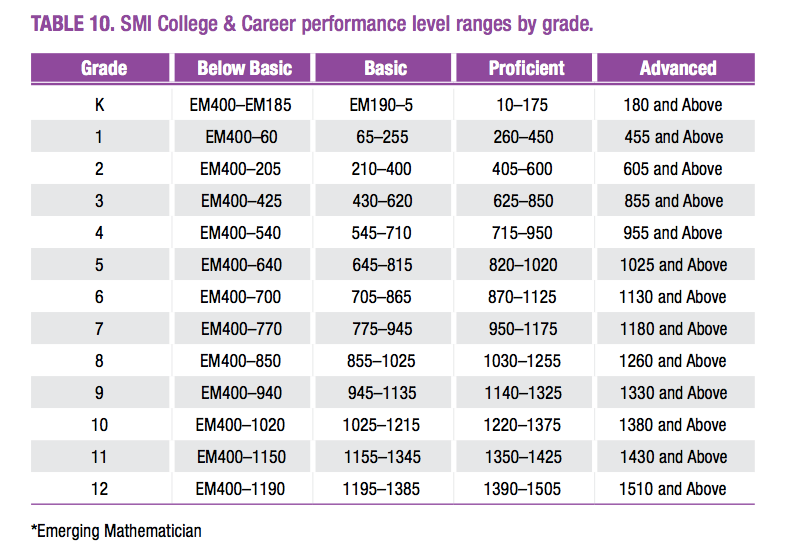• Scholastic Math Inventory: SMI

SMI Lexile Ranges Needed for a Student's Future
The Quantile level needed for Algebra Readiness is 1030Q.
The Quantile level needed for career and college pursuits measures 1400Q.

Scholastic SMI
The SRI test is measured by Quantiles.  The Quantile Framework has defined almost 500 mathematics skills and/or concepts.  Each of these concepts has a measure.  Each measure shows how difficult one skill is in relation to the others. Click here to see what skills correspond to a Quantile.
SMI test consists of age appropriate tests for K-12 students.  The test is adaptive. This means that the questions are at the student's level. The SMI test focuses on number ID, fact fluency and attending to math precision.

SMI: Quantile Resources:
www.quantile.com